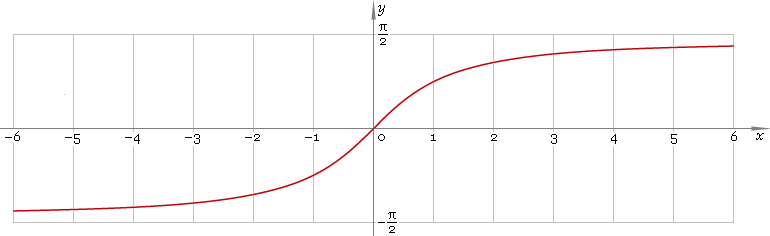The Art of Mathematics

# arctan or arctg — trigonometric arc tangent function

## 1. Definition

Arc tangent is inverse of the tangent function.

arctanxtaninvx

## 2. Graph

Arc tangent is monotone antisymmetric function defined everywhere on real axis. Its graph is depicted below in fig. 1.Fig. 1. Graph of the arc tangent function y = arctanx.

Function codomain is limited to the range (−π/2, π/2).

## 3. Identities

Complementary angle:

arctanx + arccotx = π/2

and as consequence:

arctan cot φ = π/2 − φ

Negative argument:

arctan(−x) = −arctanx

Reciprocal argument:

arctan(1/x) = arccotx for x > 0,
arctan(1/x) = arccotx − π for x < 0

Sum and difference:

arctanx + arctany = arctan[(x + y) /(1 − xy)]
arctanx − arctany = arctan[(xy) /(1 + xy)]

Some argument values:

Argument xValue arctanx
00
2 − √3π/12
√(1 − 2 /√5)π/10
√2 − 1π/8
√3 /3π/6
√(5 − 2√5)π/5
1π/4
√(1 + 2 /√5)3π/10
√3π/3
√2 + 13π/8
√(5 + 2√5)2π/5
2 + √35π/12
Table 1. Arc tangent for some argument values.

## 4. Derivative and indefinite integral

Arc tangent derivative:

arctan′x = 1 /(1 + x2)

Indefinite integral of the arc tangent:

∫ arctanx dx = x arctanx − ln(1 + x2) /2 + C

where C is an arbitrary constant.

## 5. How to use

To calculate arc tangent of the number:

``arctan(−1);``

To get arc tangent of the complex number:

``arctan(−1−i);``

To get arc tangent of the current result:

``arctan(rslt);``

To get arc tangent of the number z in calculator memory:

``arctan(mem[z]);``

## 6. Support

Trigonometric arc tangent of the real argument is supported in free version of the Librow calculator.

Trigonometric arc tangent of the complex argument is supported in professional version of the Librow calculator.